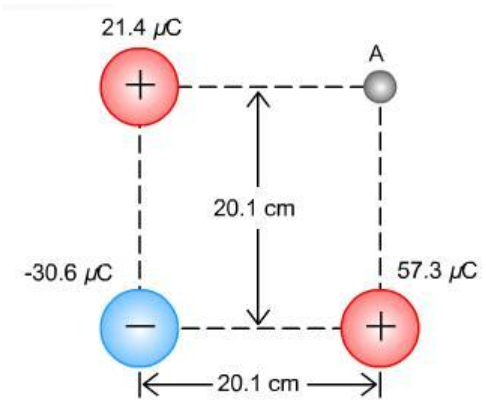# Problem: ﻿Pictured on the right are thee point charges Q1 = 21.4 μC, Q2 = -30.6 μC, and Q3 = 57.3 μC arranged according to the figure on the right. Calculate the potential at point A in the figure.

###### FREE Expert Solution

Potential:

$\overline{){\mathbf{V}}{\mathbf{=}}\frac{\mathbf{k}\mathbf{q}}{\mathbf{r}}}$

Since the potentials are scalars, we just add them directly.

We'll need to find the diagonal of the square to get r between point A and charge - 30.6μC.

H = sqrt (20.12 + 20.12) = 28.4 cm

95% (270 ratings)###### Problem Details

﻿Pictured on the right are thee point charges Q1 = 21.4 μC, Q2 = -30.6 μC, and Q3 = 57.3 μC arranged according to the figure on the right. Calculate the potential at point A in the figure.